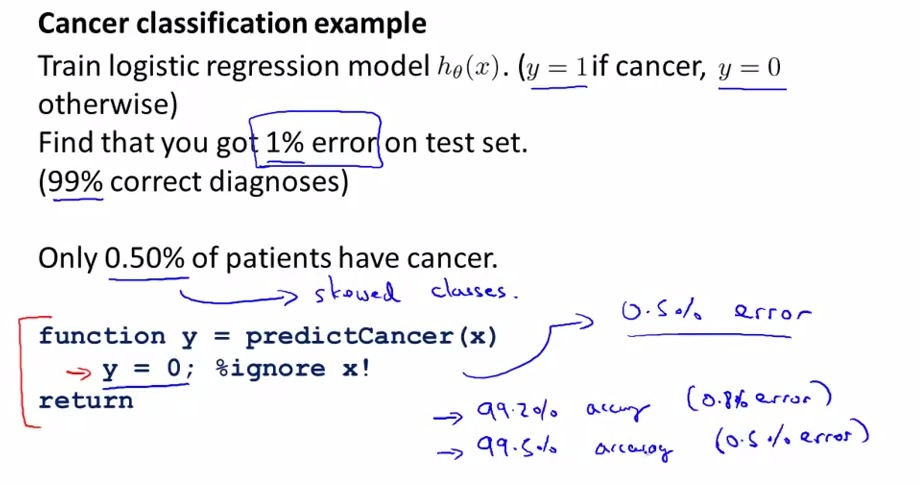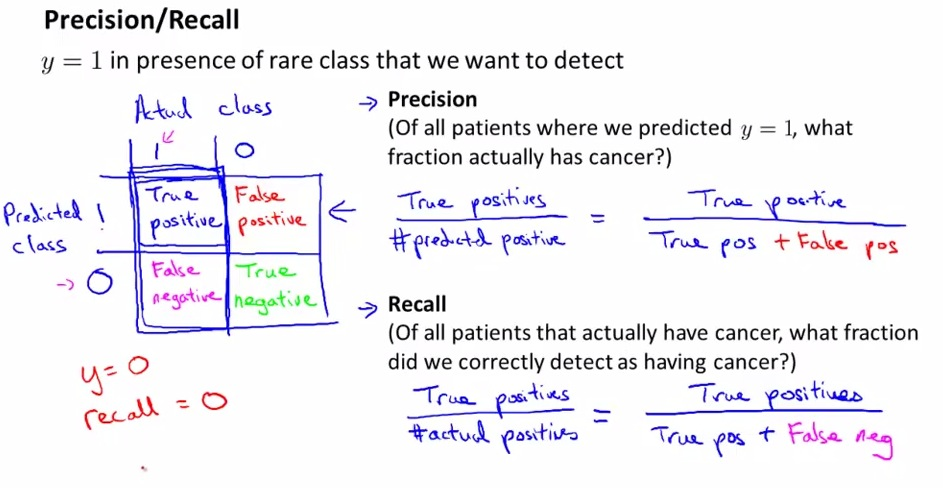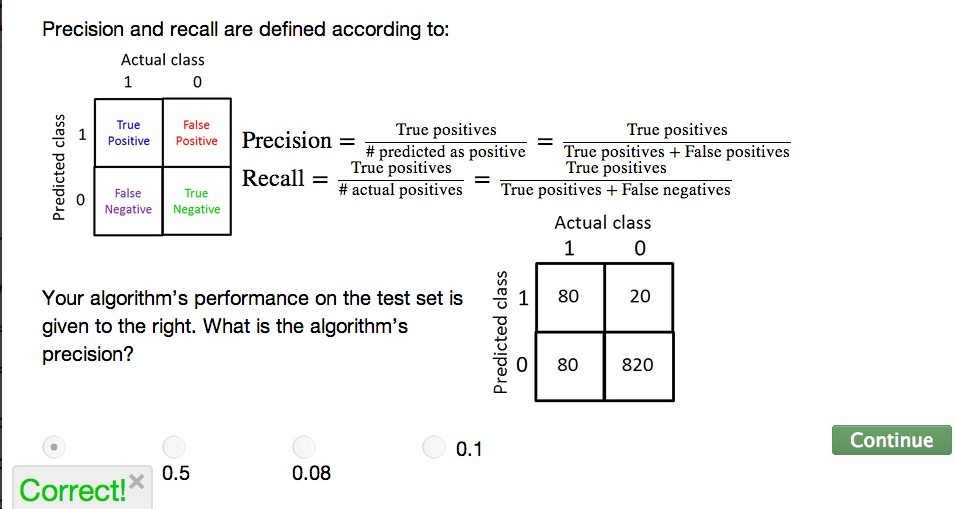Previous: error analysis, single row number of error metrics to tell how well its doing

• Skewed classes: a somewhat trickier problem to handle with error analysis.• This is cancer examples, where we have 1% error test. Suppose to be good learning algorithm?
• But as it turns out, the cancer patients only 0.50%. If we set the function that ignore X, only set y = 0 all the time(set all patients don't have cancer), then automatically we have 0.5% error test. EVEN BETTER!(sarcastically)
• If this the problem, then it is called Skewed classes. And become much harder problem.
• Skewed classes: The data that we have turns out not balance, it weight more to one class than the other
• Which turns out ignore the data is more correct than incorporating data.
• The solution? Even improving the accuracy of the algorithms, it still lack the prediction of real overall output
• Better come up with different metrics• One of different  error metrics
• Performs 2x2 table an observed whether the predicted match the actual or not
• Precision : the ratio of patients actually have cancer based on all cancer prediction (actual positive/predicted positive)
• Recall: the ratio of correctly tell them if they are indeed have a cancer. The higher the recall, the better our learning algorithm.
• Using these, in skew classes, there's no possible to cheat ( 0 or 1 all the time). For example if we set y = 0(all patients don't have cancer) all the time, then we would have recall = 0. That is we can't predict at all whether or not the patients have cancer.
• With Precision/Recall , we can tell how's the algorithm is doing well even if we have skew classes. Good error metrics for evaluation classifier for skewed classes rather than just classification error/accuracy.